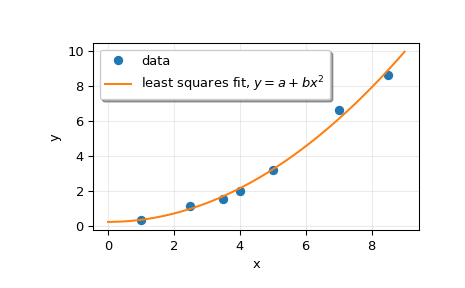# scipy.linalg.lstsq¶

scipy.linalg.lstsq(a, b, cond=None, overwrite_a=False, overwrite_b=False, check_finite=True, lapack_driver=None)[source]

Compute least-squares solution to equation Ax = b.

Compute a vector x such that the 2-norm |b - A x| is minimized.

Parameters
a(M, N) array_like

Left-hand side array

b(M,) or (M, K) array_like

Right hand side array

condfloat, optional

Cutoff for ‘small’ singular values; used to determine effective rank of a. Singular values smaller than rcond * largest_singular_value are considered zero.

overwrite_abool, optional

Discard data in a (may enhance performance). Default is False.

overwrite_bbool, optional

Discard data in b (may enhance performance). Default is False.

check_finitebool, optional

Whether to check that the input matrices contain only finite numbers. Disabling may give a performance gain, but may result in problems (crashes, non-termination) if the inputs do contain infinities or NaNs.

lapack_driverstr, optional

Which LAPACK driver is used to solve the least-squares problem. Options are 'gelsd', 'gelsy', 'gelss'. Default ('gelsd') is a good choice. However, 'gelsy' can be slightly faster on many problems. 'gelss' was used historically. It is generally slow but uses less memory.

New in version 0.17.0.

Returns
x(N,) or (N, K) ndarray

Least-squares solution. Return shape matches shape of b.

residues(K,) ndarray or float

Square of the 2-norm for each column in b - a x, if M > N and ndim(A) == n (returns a scalar if b is 1-D). Otherwise a (0,)-shaped array is returned.

rankint

Effective rank of a.

s(min(M, N),) ndarray or None

Singular values of a. The condition number of a is abs(s / s[-1]).

Raises
LinAlgError

If computation does not converge.

ValueError

When parameters are not compatible.

scipy.optimize.nnls

linear least squares with non-negativity constraint

Notes

When 'gelsy' is used as a driver, residues is set to a (0,)-shaped array and s is always None.

Examples

>>> from scipy.linalg import lstsq
>>> import matplotlib.pyplot as plt


Suppose we have the following data:

>>> x = np.array([1, 2.5, 3.5, 4, 5, 7, 8.5])
>>> y = np.array([0.3, 1.1, 1.5, 2.0, 3.2, 6.6, 8.6])


We want to fit a quadratic polynomial of the form y = a + b*x**2 to this data. We first form the “design matrix” M, with a constant column of 1s and a column containing x**2:

>>> M = x[:, np.newaxis]**[0, 2]
>>> M
array([[  1.  ,   1.  ],
[  1.  ,   6.25],
[  1.  ,  12.25],
[  1.  ,  16.  ],
[  1.  ,  25.  ],
[  1.  ,  49.  ],
[  1.  ,  72.25]])


We want to find the least-squares solution to M.dot(p) = y, where p is a vector with length 2 that holds the parameters a and b.

>>> p, res, rnk, s = lstsq(M, y)
>>> p
array([ 0.20925829,  0.12013861])


Plot the data and the fitted curve.

>>> plt.plot(x, y, 'o', label='data')
>>> xx = np.linspace(0, 9, 101)
>>> yy = p + p*xx**2
>>> plt.plot(xx, yy, label='least squares fit, $y = a + bx^2$')
>>> plt.xlabel('x')
>>> plt.ylabel('y')